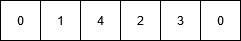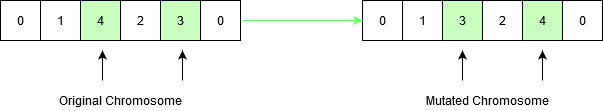# Traveling Salesman Problem using Genetic Algorithm

Prerequisites: Genetic Algorithm, Travelling Salesman Problem

In this article, a genetic algorithm is proposed to solve the travelling salesman problem.

Genetic algorithms are heuristic search algorithms inspired by the process that supports the evolution of life. The algorithm is designed to replicate the natural selection process to carry generation, i.e. survival of the fittest of beings. Standard genetic algorithms are divided into five phases which are:

1. Creating initial population.
2. Calculating fitness.
3. Selecting the best genes.
4. Crossing over.
5. Mutating to introduce variations.

These algorithms can be implemented to find a solution to the optimization problems of various types. One such problem is the Traveling Salesman Problem. The problem says that a salesman is given a set of cities, he has to find the shortest route to as to visit each city exactly once and return to the starting city.

Approach: In the following implementation, cities are taken as genes, string generated using these characters is called a chromosome, while a fitness score which is equal to the path length of all the cities mentioned, is used to target a population.

Fitness Score is defined as the length of the path described by the gene. Lesser the path length fitter is the gene. The fittest of all the genes in the gene pool survive the population test and move to the next iteration. The number of iterations depends upon the value of a cooling variable. The value of the cooling variable keeps on decreasing with each iteration and reaches a threshold after a certain number of iterations.

Algorithm:

```1. Initialize the population randomly.
2. Determine the fitness of the chromosome.
3. Until done repeat:
1. Select parents.
2. Perform crossover and mutation.
3. Calculate the fitness of the new population.
4. Append it to the gene pool.
```

## Recommended: Please try your approach on {IDE} first, before moving on to the solution.

Pseudo-code

```Initialize procedure GA{
Set cooling parameter = 0;
Evaluate population P(t);
While( Not Done ){
Parents(t) = Select_Parents(P(t));
Offspring(t) = Procreate(P(t));
p(t+1) = Select_Survivors(P(t), Offspring(t));
t = t + 1;
}
}
```

How the mutation works?

Suppose there are 5 cities: 0, 1, 2, 3, 4. The salesman is in city 0 and he has to find the shortest route to travel through all the cities back to the city 0. A chromosome representing the path chosen can be represented as:This chromosome undergoes mutation. During mutation, the position of two cities in the chromosome is swapped to form a new configuration, except the first and the last cell, as they represent the start and endpoint.Original chromosome had a path length equal to INT_MAX, according to the input defined below, since the path between city 1 and city 4 didn’t exist. After mutation, the new child formed has a path length equal to 21, which is a much-optimized answer than the original assumption. This is how the genetic algorithm optimizes solutions to hard problems.

## Recommended: Please try your approach on {IDE} first, before moving on to the solution.

Below is the implementation of the above approach:

 `// C++ implementation of the above approach ` `#include ` `#include ` `using` `namespace` `std; ` ` `  `// Number of cities in TSP ` `#define V 5 ` ` `  `// Names of the cities ` `#define GENES ABCDE ` ` `  `// Starting Node Value ` `#define START 0 ` ` `  `// Initial population size for the algorithm ` `#define POP_SIZE 10 ` ` `  `// Structure of a GNOME ` `// string defines the path traversed ` `// by the salesman while the fitness value ` `// of the path is stored in an integer ` ` `  `struct` `individual { ` `    ``string gnome; ` `    ``int` `fitness; ` `}; ` ` `  `// Function to return a random number ` `// from start and end ` `int` `rand_num(``int` `start, ``int` `end) ` `{ ` `    ``int` `r = end - start; ` `    ``int` `rnum = start + ``rand``() % r; ` `    ``return` `rnum; ` `} ` ` `  `// Function to check if the character ` `// has already occurred in the string ` `bool` `repeat(string s, ``char` `ch) ` `{ ` `    ``for` `(``int` `i = 0; i < s.size(); i++) { ` `        ``if` `(s[i] == ch) ` `            ``return` `true``; ` `    ``} ` `    ``return` `false``; ` `} ` ` `  `// Function to return a mutated GNOME ` `// Mutated GNOME is a string ` `// with a random interchange ` `// of two genes to create variation in species ` `string mutatedGene(string gnome) ` `{ ` `    ``while` `(``true``) { ` `        ``int` `r = rand_num(1, V); ` `        ``int` `r1 = rand_num(1, V); ` `        ``if` `(r1 != r) { ` `            ``char` `temp = gnome[r]; ` `            ``gnome[r] = gnome[r1]; ` `            ``gnome[r1] = temp; ` `            ``break``; ` `        ``} ` `    ``} ` `    ``return` `gnome; ` `} ` ` `  `// Function to return a valid GNOME string ` `// required to create the population ` `string create_gnome() ` `{ ` `    ``string gnome = ``"0"``; ` `    ``while` `(``true``) { ` `        ``if` `(gnome.size() == V) { ` `            ``gnome += gnome; ` `            ``break``; ` `        ``} ` `        ``int` `temp = rand_num(1, V); ` `        ``if` `(!repeat(gnome, (``char``)(temp + 48))) ` `            ``gnome += (``char``)(temp + 48); ` `    ``} ` `    ``return` `gnome; ` `} ` ` `  `// Function to return the fitness value of a gnome. ` `// The fitness value is the path length ` `// of the path represented by the GNOME. ` `int` `cal_fitness(string gnome) ` `{ ` `    ``int` `map[V][V] = { { 0, 2, INT_MAX, 12, 5 }, ` `                      ``{ 2, 0, 4, 8, INT_MAX }, ` `                      ``{ INT_MAX, 4, 0, 3, 3 }, ` `                      ``{ 12, 8, 3, 0, 10 }, ` `                      ``{ 5, INT_MAX, 3, 10, 0 } }; ` `    ``int` `f = 0; ` `    ``for` `(``int` `i = 0; i < gnome.size() - 1; i++) { ` `        ``if` `(map[gnome[i] - 48][gnome[i + 1] - 48] == INT_MAX) ` `            ``return` `INT_MAX; ` `        ``f += map[gnome[i] - 48][gnome[i + 1] - 48]; ` `    ``} ` `    ``return` `f; ` `} ` ` `  `// Function to return the updated value ` `// of the cooling element. ` `int` `cooldown(``int` `temp) ` `{ ` `    ``return` `(90 * temp) / 100; ` `} ` ` `  `// Comparator for GNOME struct. ` `bool` `lessthan(``struct` `individual t1, ` `              ``struct` `individual t2) ` `{ ` `    ``return` `t1.fitness < t2.fitness; ` `} ` ` `  `// Utility function for TSP problem. ` `void` `TSPUtil(``int` `map[V][V]) ` `{ ` `    ``// Generation Number ` `    ``int` `gen = 1; ` `    ``// Number of Gene Iterations ` `    ``int` `gen_thres = 5; ` ` `  `    ``vector<``struct` `individual> population; ` `    ``struct` `individual temp; ` ` `  `    ``// Populating the GNOME pool. ` `    ``for` `(``int` `i = 0; i < POP_SIZE; i++) { ` `        ``temp.gnome = create_gnome(); ` `        ``temp.fitness = cal_fitness(temp.gnome); ` `        ``population.push_back(temp); ` `    ``} ` ` `  `    ``cout << ``"\nInitial population: "` `<< endl ` `         ``<< ``"GNOME     FITNESS VALUE\n"``; ` `    ``for` `(``int` `i = 0; i < POP_SIZE; i++) ` `        ``cout << population[i].gnome << ``"   "` `             ``<< population[i].fitness << endl; ` `    ``cout << ``"\n"``; ` ` `  `    ``bool` `found = ``false``; ` `    ``int` `temperature = 10000; ` ` `  `    ``// Iteration to perform ` `    ``// population crossing and gene mutation. ` `    ``while` `(temperature > 1000 && gen <= gen_thres) { ` `        ``sort(population.begin(), population.end(), lessthan); ` `        ``cout << ``"\nCurrent temp: "` `<< temperature << ``"\n"``; ` `        ``vector<``struct` `individual> new_population; ` ` `  `        ``for` `(``int` `i = 0; i < POP_SIZE; i++) { ` `            ``struct` `individual p1 = population[i]; ` ` `  `            ``while` `(``true``) { ` `                ``string new_g = mutatedGene(p1.gnome); ` `                ``struct` `individual new_gnome; ` `                ``new_gnome.gnome = new_g; ` `                ``new_gnome.fitness = cal_fitness(new_gnome.gnome); ` ` `  `                ``if` `(new_gnome.fitness <= population[i].fitness) { ` `                    ``new_population.push_back(new_gnome); ` `                    ``break``; ` `                ``} ` `                ``else` `{ ` ` `  `                    ``// Accepting the rejected children at ` `                    ``// a possible probablity above threshold. ` `                    ``float` `prob = ``pow``(2.7, ` `                                     ``-1 * ((``float``)(new_gnome.fitness ` `                                                   ``- population[i].fitness) ` `                                           ``/ temperature)); ` `                    ``if` `(prob > 0.5) { ` `                        ``new_population.push_back(new_gnome); ` `                        ``break``; ` `                    ``} ` `                ``} ` `            ``} ` `        ``} ` ` `  `        ``temperature = cooldown(temperature); ` `        ``population = new_population; ` `        ``cout << ``"Generation "` `<< gen << ``" \n"``; ` `        ``cout << ``"GNOME     FITNESS VALUE\n"``; ` ` `  `        ``for` `(``int` `i = 0; i < POP_SIZE; i++) ` `            ``cout << population[i].gnome << ``"   "` `                 ``<< population[i].fitness << endl; ` `        ``gen++; ` `    ``} ` `} ` ` `  `int` `main() ` `{ ` ` `  `    ``int` `map[V][V] = { { 0, 2, INT_MAX, 12, 5 }, ` `                      ``{ 2, 0, 4, 8, INT_MAX }, ` `                      ``{ INT_MAX, 4, 0, 3, 3 }, ` `                      ``{ 12, 8, 3, 0, 10 }, ` `                      ``{ 5, INT_MAX, 3, 10, 0 } }; ` `    ``TSPUtil(map); ` `} `

Output:

```Initial population:
GNOME     FITNESS VALUE
043210   24
023410   2147483647
031420   2147483647
034210   31
043210   24
023140   2147483647
032410   2147483647
012340   24
012340   24
032410   2147483647

Current temp: 10000
Generation 1
GNOME     FITNESS VALUE
013240   21
013240   21
012430   31
012430   31
031240   32
024310   2147483647
013420   2147483647
032140   2147483647
034210   31
012430   31

Current temp: 9000
Generation 2
GNOME     FITNESS VALUE
031240   32
043210   24
012340   24
042130   32
043210   24
012340   24
034210   31
014320   2147483647
014320   2147483647
023140   2147483647

Current temp: 8100
Generation 3
GNOME     FITNESS VALUE
013240   21
042310   21
013240   21
013240   21
031240   32
013240   21
012430   31
034120   2147483647
041320   2147483647
043120   2147483647

Current temp: 7290
Generation 4
GNOME     FITNESS VALUE
031240   32
043210   24
043210   24
043210   24
012340   24
042130   32
013240   21
014320   2147483647
021340   2147483647
043210   24

Current temp: 6561
Generation 5
GNOME     FITNESS VALUE
043210   24
042310   21
042310   21
013240   21
042310   21
034210   31
013240   21
042310   21
024310   2147483647
024310   2147483647
```

GeeksforGeeks has prepared a complete interview preparation course with premium videos, theory, practice problems, TA support and many more features. Please refer Placement 100 for details

My Personal Notes arrow_drop_upCheck out this Author's contributed articles.

If you like GeeksforGeeks and would like to contribute, you can also write an article using contribute.geeksforgeeks.org or mail your article to contribute@geeksforgeeks.org. See your article appearing on the GeeksforGeeks main page and help other Geeks.

Please Improve this article if you find anything incorrect by clicking on the "Improve Article" button below.

Article Tags :
Practice Tags :

1

Please write to us at contribute@geeksforgeeks.org to report any issue with the above content.